• Total variation loss常被用在损失函数里的正则项，可以起到平滑图像，去除鬼影，消除噪声的作用 TVloss的表达式如下所示： 下面是在pytorch中的代码： import torch import torch.nn as nn from torch.autograd ...
Total variation loss常被用在损失函数里的正则项，可以起到平滑图像，去除鬼影，消除噪声的作用
TVloss的表达式如下所示：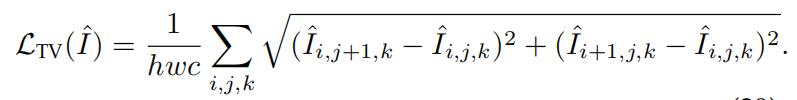下面是在pytorch中的代码：
import torch
import torch.nn as nn

class TVLoss(nn.Module):
def __init__(self,TVLoss_weight=1):
super(TVLoss,self).__init__()
self.TVLoss_weight = TVLoss_weight

def forward(self,x):
batch_size = x.size()
h_x = x.size()
w_x = x.size()
count_h =  (x.size()-1) * x.size()
count_w = x.size() * (x.size() - 1)
h_tv = torch.pow((x[:,:,1:,:]-x[:,:,:h_x-1,:]),2).sum()
w_tv = torch.pow((x[:,:,:,1:]-x[:,:,:,:w_x-1]),2).sum()
return self.TVLoss_weight*2*(h_tv/count_h+w_tv/count_w)/batch_size

def main():
x = Variable(torch.FloatTensor([[[1, 2, 3], [2, 3, 4], [3, 4, 5]], [[1, 2, 3], [2, 3, 4], [3, 4, 5]]]).view(1, 2, 3, 3),requires_grad=True)

if __name__ == '__main__':
main()



展开全文深度学习 pytorch
• 7.4 对例 7.5 进行修改，将其中的友元函数 total 改为 max，即显示三个银行中存款最 多的银行及其存款数。
#define _CRT_SECURE_NO_WARNINGS
/*
7.4 对例 7.5 进行修改，将其中的友元函数 total 改为 max，即显示三个银行中存款最
多的银行及其存款数。
*/
#include<iostream>
#include <math.h>
using namespace std;
class BBank; //这里预先说明,类 BBank 在后面说明
class GBank; //这里预先说明,类 GBank 在后面说明
class CBank //说明中国银行类 CBank
{int balance;
public:CBank() //构造函数{balance = 0;};CBank(int b) //重载构造函数{balance = b;}void getbalance(){cout << "输入中国银行存款数:";cin >> balance;}void disp(){cout << "中国银行存款数:" << balance << endl;}friend void Max(CBank, BBank, GBank);
};
class BBank //说明工商银行类 BBank
{int balance;
public:BBank() //构造函数{balance = 0;};BBank(int b) //重载构造函数{balance = b;}void getbalance(){cout << "输入工商银行存款数:";cin >> balance;}void disp(){cout << "工商银行存款数:" << balance << endl;}friend void Max(CBank, BBank, GBank);
};
class GBank //说明农业银行类 GBank
{int balance;
public:GBank() //构造函数{balance = 0;};GBank(int b) //重载构造函数{balance = b;}void getbalance(){cout << "输入农业银行存款数:";cin >> balance;}void disp(){cout << "农业银行存款数:" << balance << endl;}friend void Max(CBank, BBank, GBank);
};
void Max(CBank A, BBank B, GBank C)
{cout << "\n\n\n存款最多的是：" ;int l = 0;if (A.balance > B.balance && A.balance > C.balance){l = 1;}else if(A.balance < B.balance && B.balance > C.balance){l = 2;}else if (C.balance > B.balance && A.balance < C.balance){l = 3;}else if (A.balance == B.balance && A.balance > C.balance){l = 4;}else if (A.balance > B.balance && A.balance ==C.balance){l = 5;}else if (A.balance <B.balance && B.balance == C.balance){l = 6;}else if (A.balance == B.balance && A.balance == C.balance){l = 7;}switch (l){case 1:cout << "中国银行" <<A.balance<< endl; break;case 2:cout << "工商银行" << B.balance << endl; break;case 3:cout << "农业银行" << C.balance << endl; break;case 4:cout << "中国和工商银行一样最多" << A.balance << endl; break;case 5:cout << "中国和农业银行一样最多" << A.balance << endl; break;case 6:cout << "农业和工商银行一样最多" << B.balance << endl; break;case 7:cout << "中国，工商和农业银行一样最多" << A.balance << endl; break;default:cout << "出错了，不好意思" << endl;}
}
void main()
{CBank X(100);BBank Y;GBank Z;X.disp();Y.disp();Z.disp();//X.getbalance();Y.getbalance();Z.getbalance();Max(X, Y, Z);system("pause");
}

展开全文• //7.4 对例 7.5 进行修改，将其中的友元函数 total 改为 max，即显示三个银行中存款最 //多的银行及其存款数
//7.4 对例 7.5 进行修改，将其中的友元函数 total 改为 max，即显示三个银行中存款最
//多的银行及其存款数
#include <iostream>
using namespace std;
class BBank; //这里预先说明,类 BBank 在后面说明
class GBank; //这里预先说明,类 GBank 在后面说明
class CBank //说明中国银行类 CBank
{
int balance;
public:
CBank() //构造函数
{
balance=0;
};
CBank(int b) //重载构造函数
{
balance=b;
}
void getbalance()
{
cout << "输入中国银行存款数:";
cin >> balance;
}
void disp()
{
cout << "中国银行存款数:" << balance << endl;
}
friend void max(CBank,BBank,GBank);
};
class BBank //说明工商银行类 BBank
{
int balance;
public:
BBank() //构造函数
{
balance=0;
};
BBank(int b) //重载构造函数
{
balance=b;
}
void getbalance()
{
cout << "输入工商银行存款数:";
cin >> balance;
}
void disp()
{
cout << "工商银行存款数:" << balance << endl;
}
friend void max(CBank,BBank,GBank);};
class GBank //说明农业银行类 GBank
{
int balance;
public:
GBank() //构造函数
{
balance=0;
};
GBank(int b) //重载构造函数
{
balance=b;
}
void getbalance()
{
cout << "输入农业银行存款数:";
cin >> balance;
}
void disp()
{
cout << "农业银行存款数:" << balance << endl;
}
friend void max(CBank,BBank,GBank);
};
void max(CBank A,BBank B,GBank C)
{
cout << " 存款最多的银行是:" <<endl;
if( A.balance>B.balance&&A.balance>C.balance)
{  cout<<"CBank存款最多，且为："<< A.balance<<endl;
}
else if( B.balance>A.balance&&B.balance>C.balance)
{cout<<"BBank存款最多，且为："<< B.balance<<endl;
}
else if( C.balance>A.balance&&C.balance>B.balance)
{cout<<"GBank存款最多，且为："<< C.balance<<endl;
}
else if(A.balance==C.balance&&B.balance==C.balance) {cout<<"CBank,BBank和GBank存款一样多多，且均为："<< A.balance<<endl;
}
else if(A.balance==C.balance) {cout<<"CBank,GBank存款最多，且均为："<< A.balance<<endl;
}
else if(B.balance==C.balance) {cout<<"GBank,BBank存款最多，且均为："<< A.balance<<endl;
}
else {cout<<"CBank,BBank存款最多，且均为："<< A.balance<<endl;
}
}
int main()
{
CBank X(100);
BBank Y;
GBank Z;
X.disp();
Y.disp();
Z.disp();
//X.getbalance();
Y.getbalance();
Z.getbalance();
max(X,Y,Z);
}

展开全文• TOTAL函数系列 TOTAL函数系列共包含三个函数：TOTALMTD函数，TOTALQTD函数，TOTALYTD函数。 隶属于“值函数”，用途相对于DATESMTD系列函数更为直接。 用途：用来计算月初/季度初/年初迄今的累计值。 语法 语法1： ...TOTAL函数系列
TOTAL函数系列共包含三个函数：TOTALMTD函数，TOTALQTD函数，TOTALYTD函数。
隶属于“值函数”，用途相对于DATESMTD系列函数更为直接。
用途：用来计算月初/季度初/年初迄今的累计值。
语法
语法1：
DAX=
TOTALMTD(<表达式>,<日期>[,<筛选器>])

语法2：
DAX=
TOTALQTD(<表达式>,<日期>[,<筛选器>])

语法3：
DAX=
TOTALYTD(<表达式>,<日期>[,<筛选器>][,<结束日期>])

参数
表达式：返回标量的表达式，通常用来表示计算。
日期：日期列。
筛选器：（可选项）筛选条件。
结束日期：（可选项）用来定义年度结束日期，只有YEAR有，前两者没有。
返回结果
月初/季度初/年初至今的数据值累计之和。
例子
模拟数据：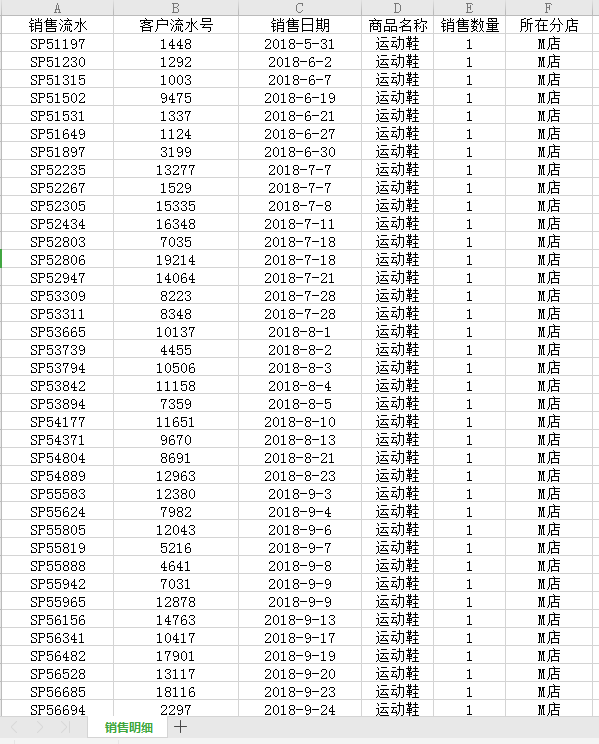这是白茶用了很多期的一份模拟数据。
例子1：
TOTALMTD例子 =
TOTALMTD ( SUM ( '销售明细'[销售数量] ), '销售明细'[销售日期])

结果：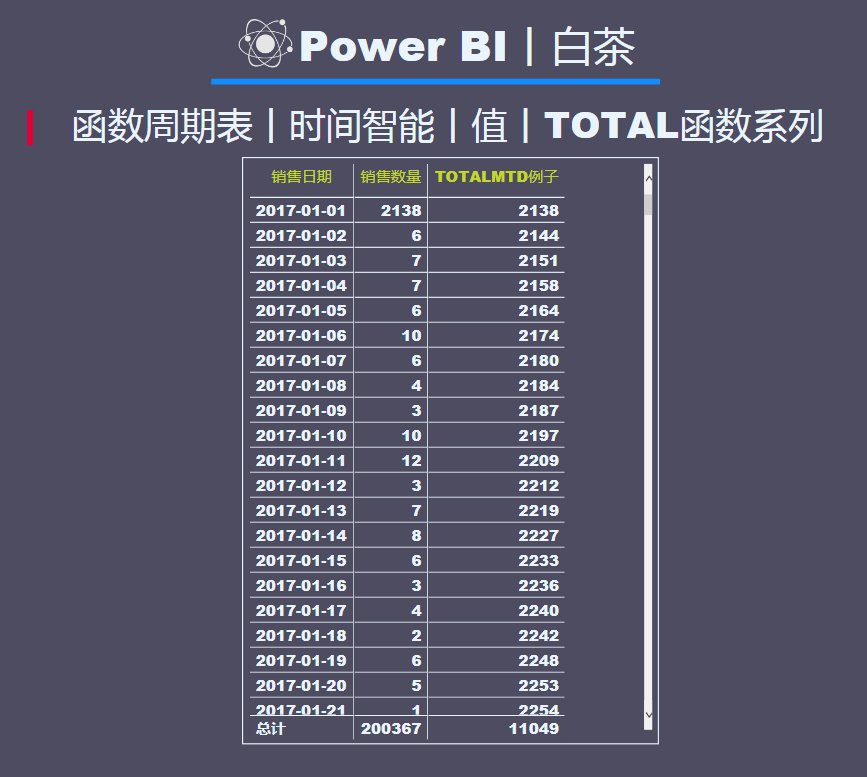按照时间维度，逐日进行累计，计算本月累计至今的数据值。
其效果等同于以下公式：
对比DATESMTD =
CALCULATE ( SUM ( '销售明细'[销售数量] ), DATESMTD ( '销售明细'[销售日期]) )

结果对比：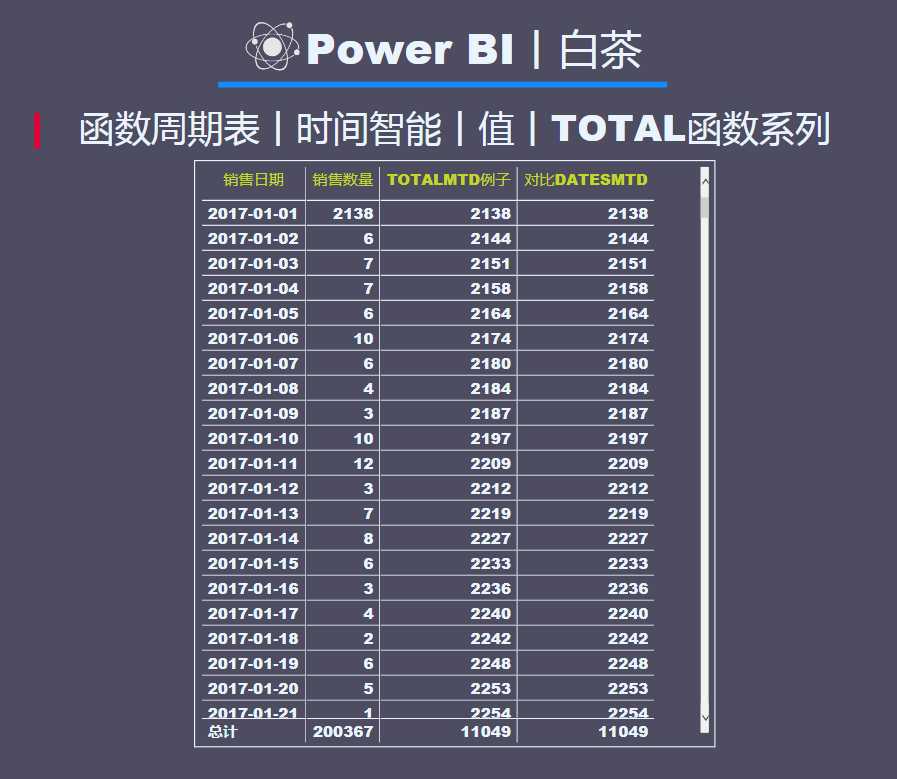可以看得出来两者的返回结果是一致的。
DATESMTD可以用来计算日期生成表，也可以与CALCULATE函数搭配求累计值。
而TOTALMTD只是单纯的计算累计值，用途更纯粹一点。
后续不再对比QTD和YTD，效果都差不多。
例子2：
TOTALQTD例子 =
TOTALQTD ( SUM ( '销售明细'[销售数量] ), '销售明细'[销售日期] )

结果：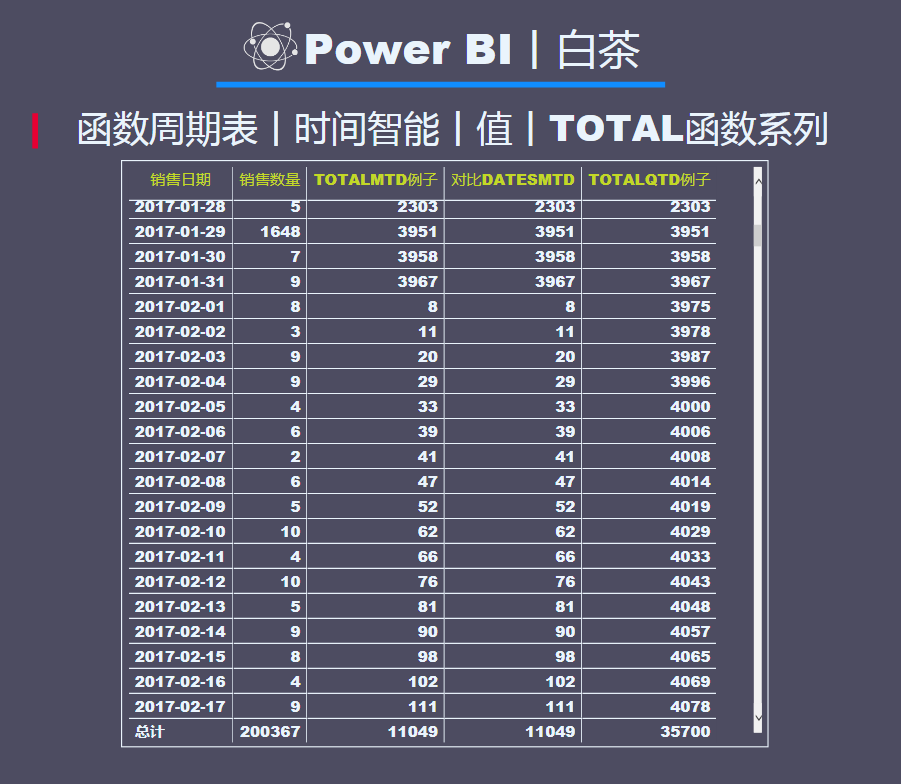逐日累计，计算本季度至今为止的累计值。
例子3：
TOTALYTD例子 =
TOTALYTD ( SUM ( '销售明细'[销售数量]), '销售明细'[销售日期] )

结果：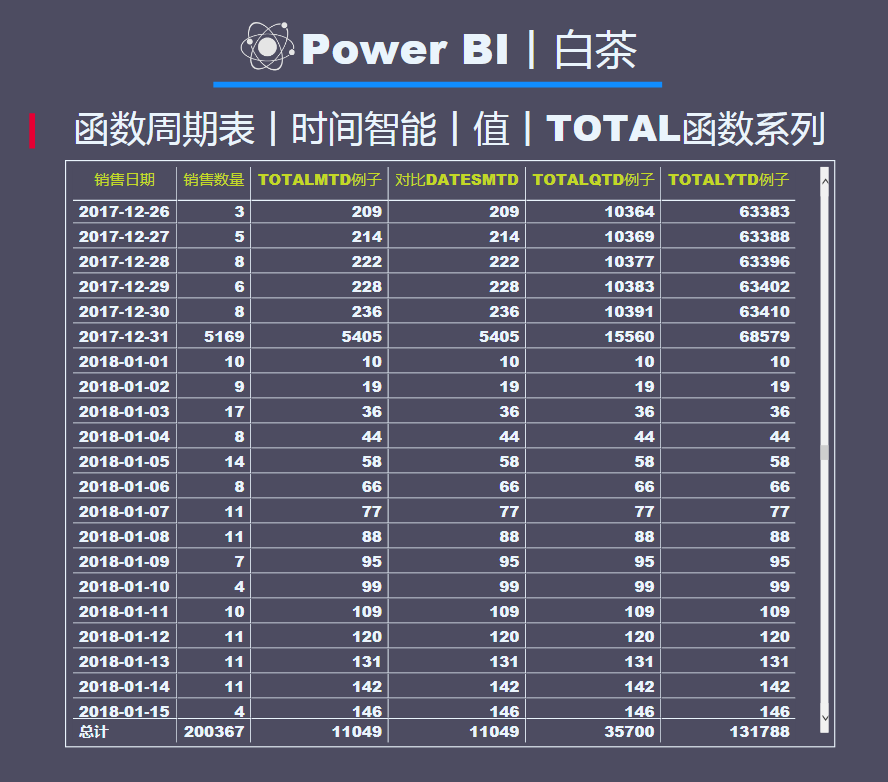逐日累计，计算本年度迄今为止的数据值累计。
到这里，时间智能函数基本上就告一段落了，对于刚开始学DAX的小伙伴来说，这一类函数作为过渡使用是再好不过的了，如果想根据具体的案例去解决具体的问题，那么还需要多多深入了解DAX哦。
小伙伴们，GET了么？
白茶会不定期的分享一些函数卡片哦。
（文件在知识星球[PowerBI丨需求圈]）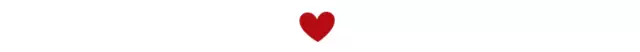这里是白茶，一个PowerBI的初学者。
下面这个知识星球是针对有实际需求的小伙伴，有需要的请加入下面的知识星球。
（这个星球里面有白茶之前所有的案例文件。）展开全文人工智能 数据分析 机器学习
• 一、TOTALYTD 释义：计算当前上下文中表达式的年初至今值 语法：TOTALYTD(表达式,日期,[筛选条件],[年末日期]) 参数： 第一参数：...USD[SalesAmount_USD]),DateTime[DateKey]) 注：TOTAL系列函数不支持DirectQuery模式power
• 一、对隐藏值的计算和忽略计算销量平均值公式：F4=SUBTOTAL(1,C2:C8)或者G4=SUBTOTAL(101,C2:C8)(1)小伙伴们有没有发现，图1中如果没有隐藏行时，两个公式的计算结果是相同的。也就相当于=SUM(C2:C8)/7(2)当图2中把...
•Cognos 实例 占比
• Total variation loss/total variation regularization/Total variation denoising ...  总变差有点像衡量一个函数变化程度的函数。BV模型一定程度上是L1图像模型。   用在图像上，total varia...
• num我们可以这样定义一个计算总销售额的函数totalSales :: num -&gt; numtotalSales 0 = 0totalSales n = totalSales (n-1) + sales (n-1)定义高阶函数total :: (num -&gt; num) -&gt;...多态 Haskell
• 解决使用mysql统计类函数为null,而不能自定义返回值的问题 SELECT COALESCE(SUM(total),0) FROM test_tablemysql select
• 查看更多： https://www.yuque.com/docs/share/922b97d2-b4e4-45bd-b567-e2616e4d1358PHP Filesystem
• 之前遇到这样的小问题，记得我之前是将图像转换为数组的形式存储，之后处理需要转换为二维的数组，大概就是这样。这里就不还原了，我用个简单的小...首先看看报错信息： **total size of new array must be unchangepython 异常处理
• 定义Boat和Car两个类，二者都有weight属性，定义二者的一个友元函数totalWeight()，计算二者的重量和。 （1） 各个类至少需包含一个构造函数、一个数据成员weight （2） 在main()中定义类的对象，...
• ## 函数

2018-07-23 18:21:10
• 1. 语句i_frame_total = p_get_frame_total( opt->hin )，实现得到输入文件的总帧数，由于p_get_frame_total = get_frame_total_yuv（见Parse（）函数），所以调用函数int get_frame_total_yuv( hnd_t handle )，在...parameters file null input
• 另有一个友元函数total用于计算储户在这三家银行中的总存款数。 CBank.cpp class BBank; class GBank; class CBank { private: double balance; public: CBank(double balance =0.0 ) { this->balan.
• {{total_price(item.goods_shop_cart)}} ... // 在计算属性中使用了闭包函数 total_num () { return function (a) { return this.goodNum(a) } }, total_price () { return function (a) { r.vue.js javascript
• 我在写一个条件分页查询时，使用mybatis-plus遇到一个问题， ...这里我使用了ifnull聚合函数结果reviewStatus 作为条件查询，分页查询时报错：在进入paginationInterceptor queryTotal找不到clounm...
• 函数 在JS当中，函数就是一个方法，(一个功能体)，基于函数一般都是为了实现某个功能，并且在后续的代码中我们依然想实现相同的操作 函数诞生的目的就是为了实现封装：把实现一个功能的代码封装到一个函数中，后期...js
• 用C++定义Boat与Car两个类，二者都有weight属性，定义二者的一个友元函数totalWeight(),计算二者的重量和 #include &lt;iostream&gt; #include &lt;bits/stdc++.h&gt; using namespace std; ...
• 匿名函数 匿名函数指一类无须定义标识符的函数或子程序。Python用lambda语法定义匿名函数，只需用表达式而无需申明。(省略了用def声明函数的标准步骤)。 #定义匿名函数： sum = lambda arg1,arg2 : arg1+arg2 #调用...
• 当类没有定义构造函数时，当对象声明时，默认构造函数调用,不用任何参数初始化 类没有构造函数，编译器假设有隐含默认的构造函数 类的对象通过简单的声明可以构造 class Example { public: int total; void ...
• 函数指针 1,如何表示指针：指针标识符 2.如何知道是函数：() 3.函数指针是专用的，格式要求很强（参数类型，个数，返回值），就像数组指针一样 #include <stdio.h> #include <stdlib.h> int ansa(int ...指针
• 用lambda关键词能创建小型匿名函数。这种函数得名于省略了用def声明函数的标准步骤。 lambda函数的语法只包含一个语句，如下： lambda [arg1 [,arg2,…argn]]:expression 如下实例： sum = lambda arg1, arg2: arg1 ...
• 实现求均值 def func2(total,count): ''' 实现求多个数据的平均值 :param total: 这个形参是指所有数据的和 :param count: 这个形参是指数据的个数 ...lambda求均值，携带参数的匿名函数 avg=l...
• 在 JS 中，函数就是一个方法，一般都是为了实现某个功能。 1. 函数的作用和创建 var total = 10; total += 10; total = total/2; total = total.toFixed(2); //=&gt; 保留小数点后面两位，toFixed 时候数字包装...
• 普通函数 def func2(total,count): ''' 实现求多个数据的平均值 :param total: 这个形参是指所有数据的和 :param count: 这个形参是指数据的个数 :return: 均值 ''' avg = total/count return avg 匿名函数 变量名=...python...